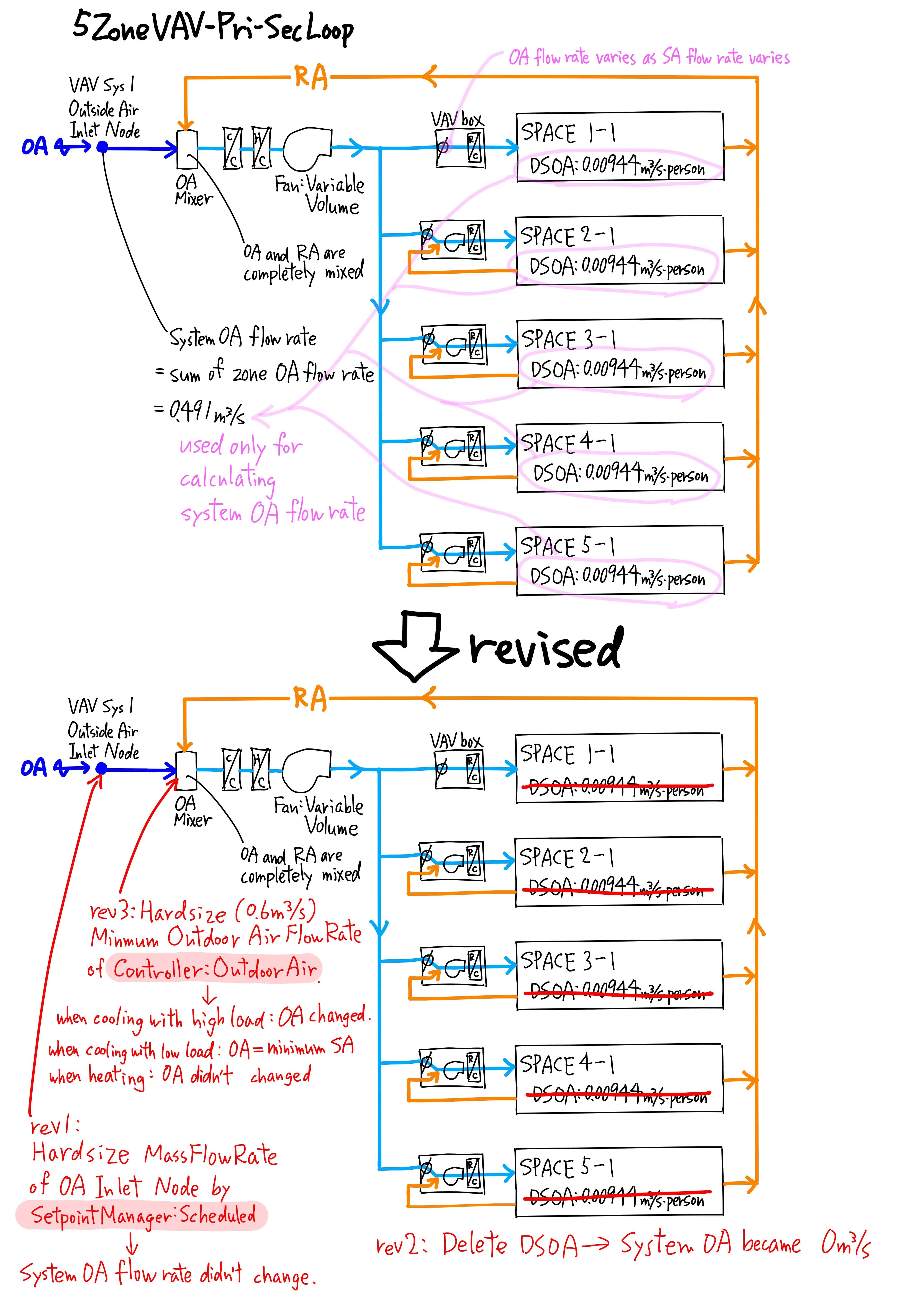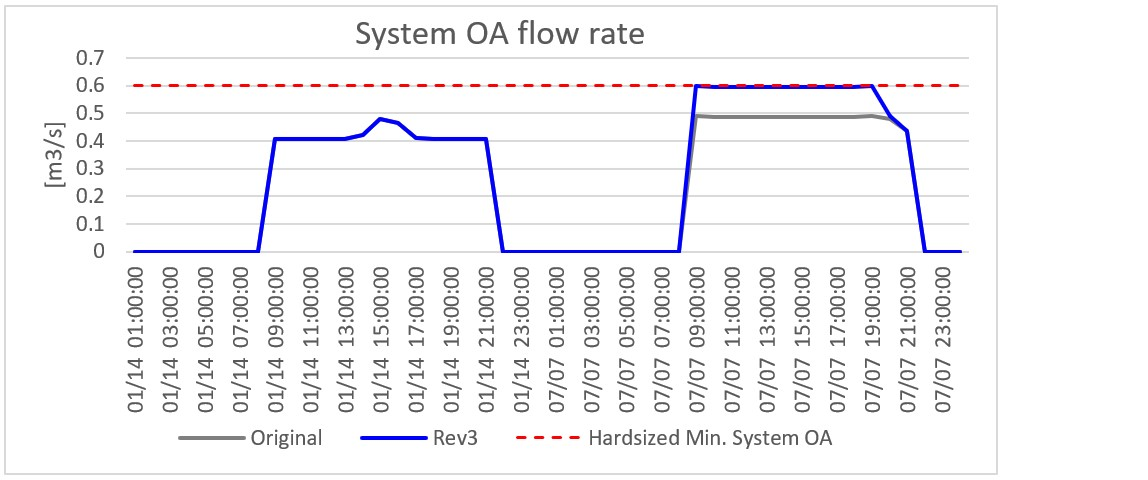# Any measure to specify System Outdoor Airflow Rate?

I there any measure to simply specify System Outdoor Airflow Rate?

When I specify the outdoor air (OA) flow rate, I use DesignSpecification:OutdoorAir (DSOA) and input DSOA objects for each space type. If there are so many zones with different DSOA, inputting all DSOAs takes time, so I'm wondering if there is any field that I can just input the total OA flow rate of the OA supply system.

For Single-Zone Systems and 100% Outdoor Air systems, we need to specify the OA flow rate for each zone. But for Multiple-Zone Sysyems (VAV systems with multiple zones), the real time OA flow rate varies since it depends on the supply air flow rate and the supply air flow rate to each zone varies according to the cooling/heating load of each zone. Only the system OA flow rate is the fixed airflow rate. The system OA flow rate is the sum of each zone OA flow rate specified by DSOA. DSOA is used only for calculating the system OA flow rate. For Multiple-Zone Systems, only the system OA flow rate matter.

I tried some things using an ExampleFile 5ZoneVAV-Pri-SecLoop.idf as an example. Below is the air-side schematic of 5ZoneVAV-Pri-SecLoop.idf. SetpointManager:Schaduled didn't work. Hardsizing Minimum Outdoor Air Flow Rate in Controller:OutdoorAir can change the system OA flow rate when cooling, but it can't change when heating. Please refer to the chart below. I couldn't find any simple and perfect way to speciy the sysyem OA flow rate.Supplement

Situation where simple hardsizing of system OA flow rate is useful: LEED submission

For ASHRAE90.1 Appendix G compliance, the OA flow rate in Baseline model should be the Minimum ventilation system outdoor air in accordance with ASHRAE 62.1. When the ventilation systems of Proposed model are Multiple-Zone Systems, we need to follow Normative Appendix A: Multiple-Zone System Ventilation Efficiency: Alternative Procedure in ASHRAE 62.1. This procedure considers the system ventilation efficiency (Ev), and the minimum system OA flow rate for Multiple-Zone Systems bacomes larger than for Single-Zone Systems and 100% Outdoor Air systems. This procedure is a bit complicated, but Minimum Indoor Air Quality Performance Calculator can calculate the minimum OA flow rate.

For LEED submission, LEED reviewers always check that the total OA flow rate for the Baseline model matches the minimum OA flow rate in this Calculator. The problem for Multiple-Zone Systems is that the Calculator doesn't calculate the minimum OA flow rate of each zone. It only calculates the system minimum OA flow rate. It can't be helped because of the calculation procedure.

Then how can we input the minimum OA flow rate to Baseline energy model? If EnergyPlus has input fields of System OA flow rate, it's super easy, but I can't find that kind of input field. For now, I estimate the minimum OA flow rates of each zone by using the system ventilation efficiency (Ev) and input them into DSOA, which ...

edit retag close merge delete